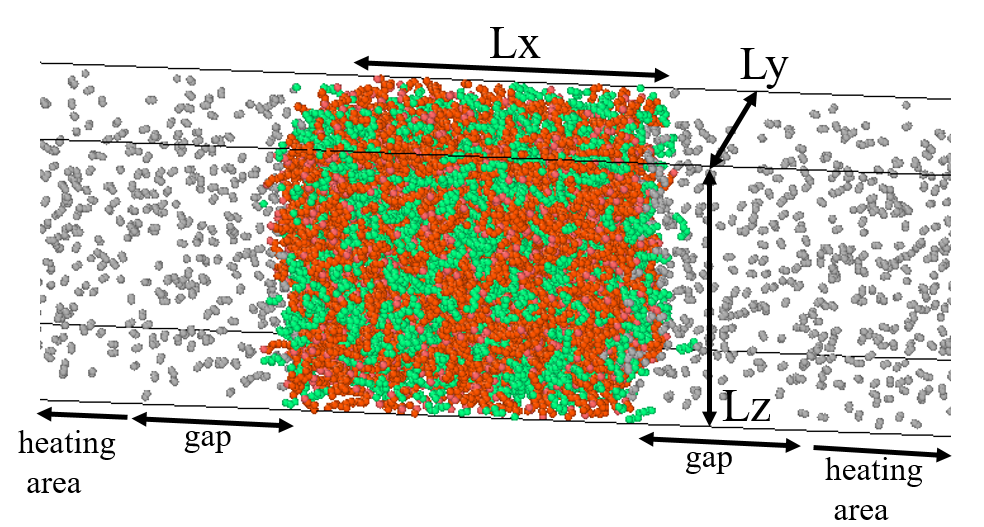# Velocity discrepancy - Kinetic theory and MD using LAMMPS

Hi All,
My simulation domain is a cuboid (image attached) with alkane (liquid) at the center and nitrogen as the ambient on either side. One of the cases, for example, involves n-heptane (at 350 K) surrounded by nitrogen at 27 bar and 350 K. When I am creating the particles, I am assigning them a velocity using:
velocity all create 350 492859 rot yes dist gaussian
velocity group_hep zero linear
velocity group_n2 zero linear
I am using the dump command to get the particle locations and velocities:
dump 1 all custom 10000 dump.atom id type x y z vx vy vz mol
Then I have a post-processing code in which I find the mean-spatial velocity variation of the particles. I was expecting that away from the interface and deep into the ambient, the nitrogen gas would follow kinetic theory formulations. But, my code gives a mean velocity of around 725 m/s and a RMS-vel of ~790 m/s. The v-RMS from kinetic theory (sqrt(3KbT/m)) on the other hand is ~570 m/s, on the other hand.
So my questions are:

1. Am I thinking on the wrong track? Is the velocity profile from MD not supposed to obey kinetic theory?
P.S.: I have even tried at low pressures of 1 bar and also on single atomic gases like helium, thinking that the shape of the molecule might have been a reason, as kinetic theory is built on assumptions of solid hard spheres as particles.
2. Is it because of the ensemble that a bias velocity is being imposed on the system at each step and that causes the higher velocity from MD? But I do a “zero linear”, shouldn’t that take care of it?
3. My MD is based in united-atom (UA) model. So I model N2 as two N atoms. So with the dump command, I get Vx, Vy and Vz of each N atom. I find the velocity magnitude (sqrt(Vx^2 + Vy^2 + Vz^2) ) for each N atom, sum them up and divide by 2 to get a velocity value for one single N2 molecule. Any flaw in this approach?

Will be hoping to get a response. Thanks in advance.please note, that there is not enough information here to make a detailed assessment of your simulation. the answers below are mostly guesses because of that.

Hi All,
My simulation domain is a cuboid (image attached) with alkane (liquid) at the center and nitrogen as the ambient on either side. One of the cases, for example, involves n-heptane (at 350 K) surrounded by nitrogen at 27 bar and 350 K. When I am creating the particles, I am assigning them a velocity using:
velocity all create 350 492859 rot yes dist gaussian
velocity group_hep zero linear
velocity group_n2 zero linear

please note, that these are all one time operations.

I am using the dump command to get the particle locations and velocities:
dump 1 all custom 10000 dump.atom id type x y z vx vy vz mol
Then I have a post-processing code in which I find the mean-spatial velocity variation of the particles. I was expecting that away from the interface and deep into the ambient, the nitrogen gas would follow kinetic theory formulations. But, my code gives a mean velocity of around 725 m/s and a RMS-vel of ~790 m/s. The v-RMS from kinetic theory (sqrt(3KbT/m)) on the other hand is ~570 m/s, on the other hand.

assuming, that you are using fix nve for time integration, this leaves the question: did you allow for equlibration of your system? depending on the initial potential energy of your system, the kinetic energy of the atoms can change quite significantly (to be higher or lower) during equilibration.

So my questions are:

1. Am I thinking on the wrong track? Is the velocity profile from MD not supposed to obey kinetic theory?

it is not clear from your system how large the space with the gas is and how the size of that space correlates with the expect mean free path of gas particles before they collide. from the picture it looks like you have a rather dense gas, so this would be on the smaller side, but that would also mean, that you can treat the system less than an ideal gas.

P.S.: I have even tried at low pressures of 1 bar and also on single atomic gases like helium, thinking that the shape of the molecule might have been a reason, as kinetic theory is built on assumptions of solid hard spheres as particles.
2. Is it because of the ensemble that a bias velocity is being imposed on the system at each step and that causes the higher velocity from MD? But I do a “zero linear”, shouldn’t that take care of it?

yes, but you are neglecting the impact of equlibration due to the choice of initial geometry. this net velocity can be easily detected by comparing the results of compute temp with compute temp/com.

1. My MD is based in united-atom (UA) model. So I model N2 as two N atoms. So with the dump command, I get Vx, Vy and Vz of each N atom. I find the velocity magnitude (sqrt(Vx^2 + Vy^2 + Vz^2) ) for each N atom, sum them up and divide by 2 to get a velocity value for one single N2 molecule. Any flaw in this approach?

no. your issues seem more on the conceptual level.

axel.